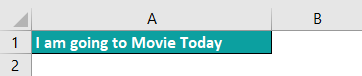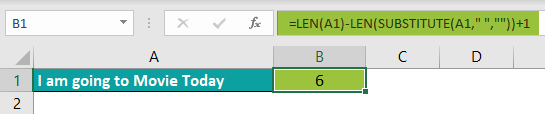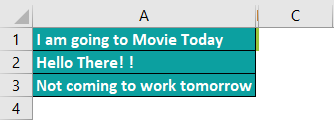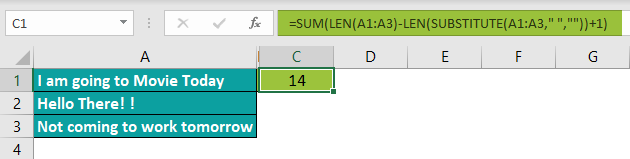# Word Count In ExcelArticle byJeevan A Y## Count Words In An Excel Cell

Excel has a variety of formulas to assist various calculations and scenarios. However, counting the words of a cell instantly is unavailable in Excel. Instead, Excel has nested formulas (example, combining LEN and SUBSTITUTE) that we can use to count words.

Counting words refers to the number of words in a cell. For instance, consider the following example. In cell A1, we have the word ‘How are you.’

The number of words in cell A1 is 3, i.e., the word count is 3.

This is how the word count function works.

###### Key Takeaways
• There is no in-built function to count the number of words in a cell or range of cells in Excel.
• We can get the total word count by combining the LEN and SUBSTITUTE functions from a single cell. However, to get the word count from multiple cells, we must wrap these functions with the SUM or SUMPRODUCT function.
• Excel treats the text as a word after every space character.
• To handle the blank rows, we need to use the IF condition.

### How To Count The Total Number Of Words In A Range Of Cells?

In the Excel formulas library, we have a TEXT function category. Here, we have many formulas to deal with text or string values in Excel.

One such formula is the LEN Excel function.

LEN function helps us to get the number of characters in a cell. When we say the number of characters, it is not the number of words but the number of characters.

For instance, look at the following value in a cell.

Step 1: Enter the LEN function in cell B1.

Step 2: For the LEN function, we need to choose the cell from which we need to count the number of characters, i.e., cell A1.

The total number of characters in cell A1 is 11. However, when we count the number of characters, it is only 9, not 11.

LEN function treats space as a character, and in the above cell A1 we have 2 spaces between each word, so the count, i.e., the total characters = 11.

Please Note:  We have introduced you to this function because we will be counting the number of words in a cell using this function.

Let us look at the following example to understand the method.

#### Example #1: Count Words in a Single Cell

The below table consists of a few conversations in column A. But, first, let us learn how to do word count in Excel.

The steps used to count words are as follows:

Step 1: First, by using the LEN function, let us count the number of characters from the above cells.

Step 2: The total number of characters are 11.

Next, we need to count the characters without the space.

For this, we need to use the SUBSTITUTE function.

Step 3: The first argument of the SUBSTITUTE function is text, i.e., the text we need to substitute. So, choose cell A2.

Step 4: The old text we need to substitute is the space character, so enter the space character in double-quotes.

Step 5: Next, the new text argument in this example will be empty since we do not have anything to substitute with the old text.

So, we need to leave the argument by simply entering double quotes.

Step 6: Close the bracket and hit the enter key to get the new text value.

Step 7: We have got the new text without any space characters. However, we do need the text, but we need only the character count without spaces. Hence, wrap the SUBSTITUTE function with the LEN function.

Step 8: The total character count is 9 without the space character. To get the total word count, get the difference between character count with space and without space, i.e., 11-9

Step 9: The total word count is 2, but the actual word count is 3.

So, we are getting 1 less.

To fix this issue, add +1 to the eventual result.

Now, the total word count is 3.

Step 10: However, instead of following a multi-step process, we can combine all the steps in a single step. First, copy the combination formula to SUBSTITUTE and LEN functions and paste it inside the first formula with a minus sign.

Step 11: Copy the formula to all the below cells and see the result.

The last result shows the word count as 3 even though we have only 2 words in cell A5.

Step 12: The reason for this is the extra space character before the start of the word in cell B5.

Hence, whenever there is any extra unnecessary space character, we get a different word count with the above method.

Step 13: We need to use the TRIM excel function for the first LEN function to tackle these scenarios.

The TRIM function will remove all the unnecessary space and returns only the simple text to the LEN function.

Step 14: However, the above function will give us a word count as 1 whenever we have a blank cell as shown below.

Step 15: In cell A5, we do not have any values, but still, we got the result as 1.

So, to deal with these scenarios, we need to include the IF condition.

In the above formula, the IF condition checks for the cell first. If the cell is blank, it will return 0, or it will execute the word count formula.

#### Example #2: Count Words in Range of Cells

In the above example, we have seen counting words from a single cell. Let us learn how to do word count in excel from multiple cells and get the total sum of word count.

We need to use the SUM excel function to get the sum of words from the multiple cells.

Step 1: The formula looks the same as the previous one, but this time select the range of cells rather than a single cell reference.

Step 2: Instead of selecting one cell, we have selected the range of cells as A2:A5, and then the SUM function will return the sum of all the words.

The total word count in a range of cells from A2:A5 is 15. So, as we can see, it also matches with the individual cell’s word count.

Note: If you are using Excel 2016 and prior versions of Excel, you need to close the formula as an array formula. You must hit the enter key by holding the Ctrl + Shift key together.

Instead of the SUM function, we can also use the SUMPRODUCT function.

### Count Specific Words In A Range

In our earlier examples, we have seen counting words in a single cell and counting words from the range of cells. Now let us look at the example of counting only specific word count in excel words from a cell or range of cells.

For instance, we have the following data in Excel.

Assume we need to count only a specific word, say ‘Monday’

The steps used to find specific word count in excel are as follows:

Step 1: Create a cell reference for the word we need to count. In our case, we have entered Monday in cell B1.

Step 2: First, find the total number of characters in a cell using the LEN function.

The total number of characters in cell A2 is 61.

Step 3: Next, we need to replace the word ‘Monday’ with the empty string using the SUBSTITUTE function.

Step 4: After removing the word ‘Monday’ from the text, we need to get the total count of characters, so wrap the SUBSTITUTE function with the LEN function.

Step 5: Get the difference between these two character counts.

Step 6: Now get the total number of characters in the word we are searching for, i.e., ‘Monday.’

Step 7: Now divide the difference and total characters of the targeted word to get the targeted word count.

So, the total word count for the word ‘Monday’ in cell A2 is 2.

Step 8: Since this is a lengthy process, let us combine all the formulas in a cell to avoid multiple steps.

Step 9: Copy the formula to all the below cells to get the word count of Monday in each cell.

Similarly, to get the word count of all cells in a single cell, we can use either the SUM function or SUMPRODUCT function.

Likewise, we can find specific word count in excel with a few steps.

### Count Repeated Words In Excel

In our earlier examples, we have seen counting all the words and specific words. Now let us learn how to do word count in excel for repeated words.

Look at the following data in Excel.

We have the salesperson’s name and the city they belong to. Now we need to count the city names repeating more than once.

To do this, first, we need to find what cities are repeating by using the COUNTIF and IF Excel function.

In the above formula, COUNTIF will search for the particular city count in the range B2:B10. If the count is more than 1, then the IF condition will return the word ‘Repeating,’ or it will return the blank cell.

Now apply another COUNTIF function to count the ‘Repeating’ word.

The total word count of the words which are repeated is 5.

In this way, we can find word counts in Excel.

### Important Thing To Note

• The LEN function treats the space as a character.
• The TRIM function is used to remove all the unwanted space characters.
• While using Excel 2016 and prior versions, we need to close the SUM function with Ctrl + Shift + Enter to close the formula as an array function.
• The SUBSTITUTE is a case-sensitive function, so we must be careful in supplying the word to this function. For instance, the words ‘hello’ and ‘Hello’ are not the same in the SUBSTITUTE function.

How to do word count in Excel?

Word count in excel is a simple process. For instance, consider the following excel sheet with text string. Now, we need to use the following steps to check word count in excel.Enter the LEN and SUBSTITUTE functions in cell B1 with the arguments.

Press Enter key.

Excel will return the word count as shown in the image below.How to find word count in multiple cells in Excel?

Counting words in multiple cells is simple. For instance, consider the below table with data in multiple cells. But, first, learn to check word count in excel for multiple cells.To get the word count from all these cells, we need to select the cell as an array for LEN and SUBSTITUTE functions and then wrap the functions with the SUM of the SUMPRODUCT function.What is the Function or Formula for Word Count in Excel?

We do not have any built-in function to get the word count in Excel. We need to use text functions such as LEN and SUBSTITUTE as a combo to get the word count.

However, if we are looking for the word count from more than one cell, we need to choose all the range of cells as an array and then surround these functions with the SUM or SUMPRODUCT function.